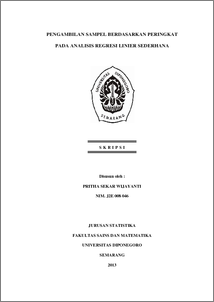# PENGAMBILAN SAMPEL BERDASARKAN PERINGKAT PADA ANALISIS REGRESI LINIER SEDERHANA

WIJAYANTI, PRITHA SEKAR (2013) PENGAMBILAN SAMPEL BERDASARKAN PERINGKAT PADA ANALISIS REGRESI LINIER SEDERHANA. Undergraduate thesis, UNIVERSITAS DIPONEGORO.Preview
PDF
1773Kb

## Abstract

Ranked Set Sampling and Ranked Set Sampling concomitant are more efficient than Simple Random Sampling. This can be determined by calculating the Relative Precision which is a ratio value from the variance of the mean from each sampling technique. From the research of Ranked Set Sampling, obtained Var(Y ̅_[m]r')<Var(Y ̅'), RP'>1 and RS'>0 so Ranked Set Sampling is more efficient than Simple Random Sampling. For the research of Ranked Set Sampling concomitant, obtained Var(Y ̅_X(m)r')<Var(Y ̅'), 〖RP〗_Y^c'>1, 〖RS〗_Y^c'>0 so Ranked Set Sampling concomitant is more efficient than Simple Random Sampling, and for simple linear regression analysis obtained Var(β ̂_0(RSS) ')<Var(β ̂_0(SRS) '), Var(β ̂_1(RSS) ')<Var(β ̂_1(SRS) '), 〖RP〗_(β ̂_0 )'>1, 〖RP〗_(β ̂_1 )'>1 so simple linear regression model of Ranked Set Sampling is more efficient than simple linear regression model of Simple Random Sampling. Keywords : Ranked Set Sampling, Ranked Set Sampling Concomitant, Relative Precision, Simple Linear Regression Analysis

Item Type: Thesis (Undergraduate) H Social Sciences > HA Statistics Faculty of Science and Mathematics > Department of Statistics 42505 Mr Hasbi Yasin 25 Feb 2014 16:14 25 Feb 2014 16:14

Repository Staff Only: item control page